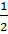### Sample Problem

Which is the correct graph of y =|x – 1| + 1?

#### Solution

The coefficient is, so the graph should be twice as wide as y = |x|. This leaves us with choices (B) and (C). (C) is shifted 1 unit to the left, but the expression |x – 1| calls for a shift to the right, so (B) must be the correct answer.